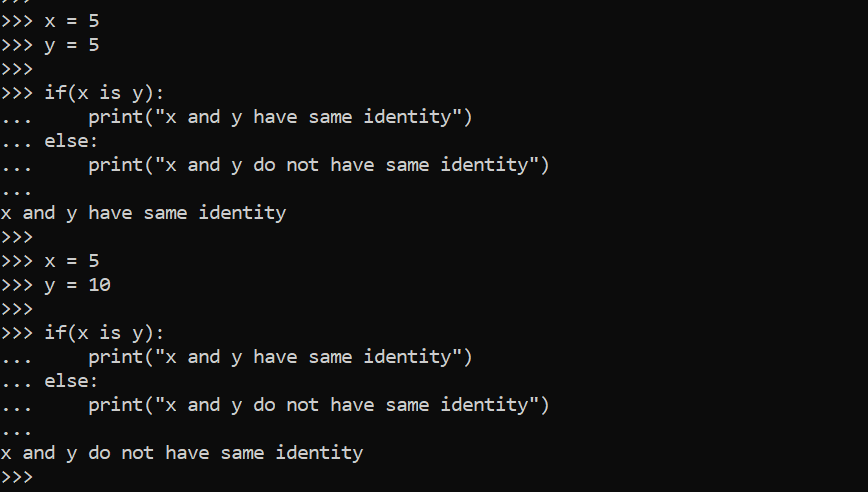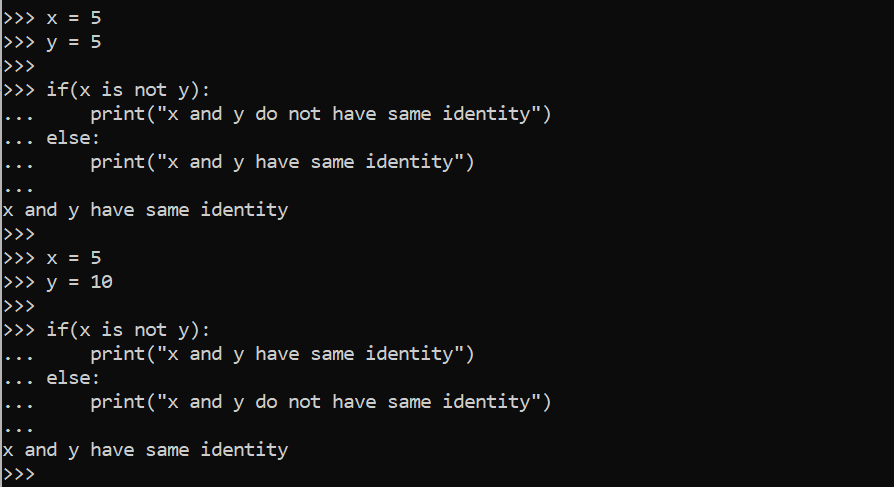Scripting

# Identity Operators in PythonIdentity operators in Python are used to determine if a value belongs to a specific class or type and are frequently utilized to figure out what kind of data a variable contains. Identity operators, as their name implies, are used to locate an object’s memory unit, particularly when two objects have the same name and can only be distinguished by their memory location.

## Types of Identity Operators in Python

There are two different types of identity operators listed below

• Is operator
• Is Not operator

## Python Identity operators with examples

There are two main identity operators, which are the “is” operator and the “is not” operator, that will be discussed in this article.

## Is Operator in Python

When two variables point to the same object or have the same value, the “is” operation returns true; otherwise, it returns false.

### Example 1:

 ```x = 5 y = 5 print(x is y) Output: True x = 5 y = 10 print(x is y) Output: False```We are utilizing two variables, “x” and “y” in the preceding example of the “is” operator and giving them the same values, as x = 5 and y = 5 implies the same memory address or refers to the same object. In the second example, you can see that their values are 5 and 10, which are not the same, which is why the result is false.

### Example 2:

In this example, we are going to use if-else statements for better understanding the same variables such as x and y and assign them values to differentiate them from each other.

 ```x = 5 y = 5 if ( x is y ): print("x and y have same identity") else: print("x and y do not have same identity") Output: x and y have same identity x = 5 y = 10 if ( x is y ): print("x and y have same identity") else: print("x and y do not have same identity") Output: x and y do not have same identity```
``

## Is Not Operator in Python

Is Not operator works in the opposite direction to the operator. If two objects have different memory locations, this returns true. If two objects or variables have the same memory location, this returns False.

### Example 1:

 ```x = 5 y = 10 print(x is not y) Output: True x = 10 y = 10 print(x is not y) Output: False```As you can see in the example of the “is not” operator we are using two variables, “x” and “y” and assigning them different values, as x = 5 and y = 10 means they have different memory locations or are pointed to a different object. As their values are not the same, in this case, the output will be true, whereas you can see that in the second example, the value is 5 for each variable, which is the same that’s why the output will be false

### Example 2:

Same as the above example of “is” operator we are going to use if-else statements for better understanding of the “is not” operator with the same variables such as x and y and assigning them values to differentiate the memory location or object of variables from each other.

 ```x = 5 y = 5 if ( x is not y ): print("x and y have same identity") else: print("x and y do not have same identity") Output: x and y do not have same identity x = 5 y = 10 if ( x is not y ): print("x and y have same identity") else: print("x and y do not have same identity") Output: x and y do not have same identity```
``

In this example, as you can see, we used condition statements “if-else” with the “is not” operator to identify the variable’s memory location, whether they are pointing to the same object or not, or whether they are identical or not. In the above example if the condition is True, it returns the result both “x and y have the same identity” otherwise it returns “x and y do not have the same identity”.

## Conclusion

In Python, identity operators are used to determine if a value belongs to a given class or type, and they are typically used to identify what sort of data a variable contains. Identity operators are used to find an object’s memory unit, which is especially useful when two objects have the same name and can only be identified by their memory location.